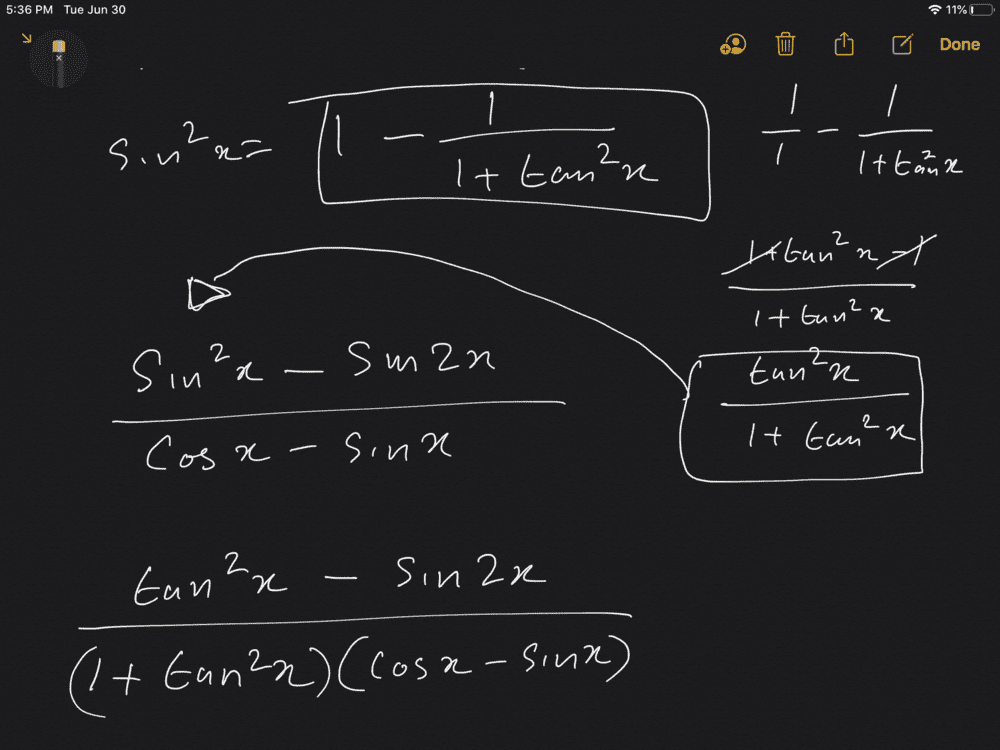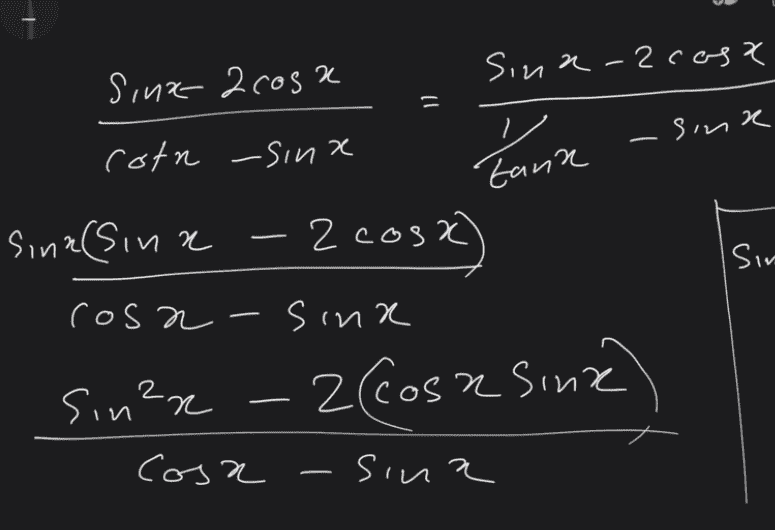# Evaluate this trigonometric identity

lioric
Your expression is still not right, since you didn't distribute the ##\sin{x}## to both terms in the denominator.

If you still want to do it the long way, you need to find ##\sin{x}## and ##\cos{x}## in terms of ##\tan{x}##. To get you started,

$$\sec^2{x} = 1+\tan^2{x}$$ $$\cos^2{x} = \frac{1}{1+\tan^2{x}}$$ $$\sin^2{x} = 1- \frac{1}{1+\tan^2{x}}$$

Simplify those, N.B. you need to use the quadrants to figure out the signs after you take the square root.
Thank you very much

lioric
Your expression is still not right, since you didn't distribute the ##\sin{x}## to both terms in the denominator.

If you still want to do it the long way, you need to find ##\sin{x}## and ##\cos{x}## in terms of ##\tan{x}##. To get you started,

$$\sec^2{x} = 1+\tan^2{x}$$ $$\cos^2{x} = \frac{1}{1+\tan^2{x}}$$ $$\sin^2{x} = 1- \frac{1}{1+\tan^2{x}}$$

Simplify those, N.B. you need to use the quadrants to figure out the signs after you take the square root.
I know you might say
” I already told this guy how to do this. Why wouldn’t he just go and finish the question the way we taught him”
its just that I see how lot of you guys simply with such ease, and I also know that there are several methods to doing one question, and I just feel I’d understand the concept better when I do it from different methods to converge on the answer. So please don’t be frustrated with me.
i thank you for your patience with me

I know you might say
” I already told this guy how to do this. Why wouldn’t he just go and finish the question the way we taught him”
its just that I see how lot of you guys simply with such ease, and I also know that there are several methods to doing one question, and I just feel I’d understand the concept better when I do it from different methods to converge on the answer. So please don’t be frustrated with me.
i thank you for your patience with me

Well sure, but the idea is that given enough time to google the formulae and do the algebra I'm pretty sure you would be able to get the answer the long way. It's more helpful for you to learn more efficient ways to solve things like this.

lioric
Well sure, but the idea is that given enough time to google the formulae and do the algebra I'm pretty sure you would be able to get the answer the long way. It's more helpful for you to learn more efficient ways to solve things like this.
I know
i just have a hard time figuring out which goes where.

lioric
Just to clarify, am I on the right track so far?You still haven't distributed the ##\sin{x}## into the denominator

Last edited by a moderator:
Homework Helper
Gold Member
2022 Award
Just to clarify, am I on the right track so far?
View attachment 265540
You were better off with the function you started with. Generating ##\sin(2x)## is a step in the wrong direction altogether.

•etotheipi
Yes I'm not even sure why you would want to multiply by ##\frac{\sin{x}}{\sin{x}}## in the first place

lioric
ok ppl
let me start off again

lioric
Your expression is still not right, since you didn't distribute the ##\sin{x}## to both terms in the denominator.

If you still want to do it the long way, you need to find ##\sin{x}## and ##\cos{x}## in terms of ##\tan{x}##. To get you started,$$\sec^2{x} = 1+\tan^2{x}$$ $$\cos^2{x} = \frac{1}{1+\tan^2{x}}$$ $$\sin^2{x} = 1- \frac{1}{1+\tan^2{x}}$$Simplify those, N.B. you need to use the quadrants to figure out the signs after you take the square root.
Wait so are you saying that I have to root these things, and use the quadrants, find the value and signs, and substitute it into the main question?
It's pretty much the same as the triangle version right?

It's pretty much the same as the triangle version right?

You betHomework Helper
Gold Member
2022 Award
Wait so are you saying that I have to root these things, and use the quadrants, find the value and signs, and substitute it into the main question?
It's pretty much the same as the triangle version right?
You can plug those things in and try to simplify. Maybe you get a nice simple answer and maybe you don't. I suspect that in this case you won't get a nice simple formula in terms of ##\tan x##.

•lioric
lioric
So there is no way to do this without plugging values right?
I was thinking of simplify it without any values
But as you said we need the values to proceed. So just like the triangle. Thank you very much.

lioric
Everytime I come here, my mind flourishes. I'd like to thank all the people here who help people like us to get our brains thinking like that

•Delta2 and berkeman
Homework Helper
Gold Member
Even if you can find trig identities to solve the problem, that is not what the problem was expecting you to do. The solution where you use the 3,4,5 right triangle (or determine an angle from trig tables) is the fundamental thing to learn and what the problem expected. Do not go down the "rabbit hole" of trig identities when that is not necessary.

•Delta2
Staff Emeritus
Homework Helper
Gold Member
Homework Statement:: If tanx = 4/3 and π < x < 3π/2 , evaluate the following
(Sinx-2cosx) / (cotx-sinx)
Relevant Equations:: Tan x=sinx/cosx
Cotx=1/tan
One of the difficulties you have stems from incorrect algebra.
(Sinx-2cosx)/ (cotx - sinx)
You did this fine in your "blackboard" image. You get:

## \displaystyle \frac{\sin x - 2\cos x}{\dfrac{1}{\tan x}-\sin x} ##
(Tanx(sinx-2cosx)/(1-sinx)
That result is incorrect. Presumably you are multiplying the numerator and denominator by tan x , but you didn't distribute tan x through the denominator. Distributing correctly gives:

## \displaystyle \frac{\tan x (\sin x - 2\cos x)}{1 - \tan x \cdot \sin x} ## , which you might as well write as:

## \displaystyle \tan x \ \ \frac{\sin x - 2\cos x}{1 - \tan x \cdot \sin x} ##

This gets you close to what @PeroK has been suggesting. Just multiply numerator and denominator by sec(x), also known as 1/cos(x) .

Last edited:
•vela
lioric
One of the difficulties you have stems from incorrect algebra.
You did this fine in your "blackboard" image. You get:

## \displaystyle \frac{\sin x - 2\cos x}{\dfrac{1}{\tan x}-\sin x} ##
That result is incorrect. Presumably you are multiplying the numerator and denominator by tan x , but you didn't distribute tan x through the denominator. Distributing correctly gives:

## \displaystyle \frac{\tan x (\sin x - 2\cos x)}{1 - \tan x \cdot \sin x} ## , which you might as well write as:

## \displaystyle \tan x \ \ \frac{\sin x - 2\cos x}{1 - \tan x \cdot \sin x} ##

This gets you close to what @PeroK has been suggesting. Just multiply numerator and denominator by sec(x), also known as 1/cos(x) .
That made a lot of sense. I was trying to simply do the reciprocal since it was 1/tan. But the -sinx is the issue here.
Thank you very much

Staff Emeritus
Homework Helper
Gold Member
That made a lot of sense. I was trying to simply do the reciprocal since it was 1/tan. But the -sinx is the issue here.
Thank you very much
I'm not sure what you mean by:
−sin x is the issue here.

In post #33 you had posted two images. Here's a snip from the first:The last line there should be ##\dfrac{\sin^2 x - 2\sin{x}\cos x}{\cos{x} - \sin^2{x}}##, which is equivalent to what @etotheipi posted in Post #27.

Presumably, you intended to multiply the numerator and denominator of original expression by sin x , but you failed to distribute that in the denominator.

Algebra !

•DifferentialGalois and vela
Homework Helper
Homework Statement:: If tanx = 4/3 and π < x < 3π/2 , evaluate the following
(Sinx-2cosx) / (cotx-sinx)
Relevant Equations:: Tan x=sinx/cosx
Cotx=1/tan

(Sinx-2cosx)/ (cotx - sinx)
(Tanx(sinx-2cosx)/(1-sinx)
What do I do from here
I don't think what I did there is correct
That's why I didn't expand the tan to sin/cos
You could divide through by cos(x) and then most of the terms would be known from the given(s). Just need one additional ratio which you can extract via Pythagoras thm (and quadrant data) as others have advised.

•SammyS
Homework Helper
Gold Member
Fully aware that too many cooks spoil the broth, I will summarize a straightforward solution based on statements that have been by others, but is independent of the ratio of the sides of the implied right triangle. My intent is to help wrap up this thread.
1. Let ##u=\tan x##.
2. Because angle ##x## lies in the third quadrant, both ##\sin x## and ##\cos x## are negative. We write$$\sin x=-\frac{u}{\sqrt{1+u^2}}~;~~~\cos x=-\frac{1}{\sqrt{1+u^2}}.$$We can deduce these expressions by noting that the sum of their squares must be ##1## and that their ratio must be ##u.##
3. Use the given value ##u=\frac{4}{3}## to find numerical values for the sine and cosine and substitute them in the expression remembering that two negative signs make a positive sign.
4. Simplify the fraction to a ratio of integers.

Homework Helper
From "chef WA"and @SammyS I must admit the suggestion I made came from WA in the first place.